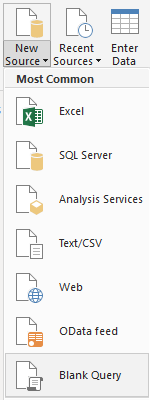Below is the syntax to create a Time Dimension Table in Power Query

I had a requirement where I needed to create a Time Dimension for a customer. Most of the time I only need the date. Upon searching I could not find a resource where they had created the time dimension only using Power Query.

• What I did was I opened my Power BI Desktop file and went into the Query Editor
• I then clicked on Get Data and selected Blank Query
•• I renamed the Query to Time
• I then clicked on the Advanced Editor to allow me to paste in my code
•• I then pasted in the code below
```let
Source = List.Times(#time(0, 0, 0), 1440, #duration(0, 0, 1, 0)),
#"Converted to Table" = Table.FromList(Source, Splitter.SplitByNothing(), null, null, ExtraValues.Error),
#"Renamed Columns" = Table.RenameColumns(#"Converted to Table",{{"Column1", "Time"}}),
#"Changed Type" = Table.TransformColumnTypes(#"Renamed Columns",{{"Time", type text}}),
#"Duplicated Column" = Table.DuplicateColumn(#"Changed Type", "Time", "Time - Copy"),
#"Split Column by Delimiter" = Table.SplitColumn(#"Duplicated Column", "Time - Copy", Splitter.SplitTextByEachDelimiter({":"}, QuoteStyle.Csv, false), {"Time - Copy.1", "Time - Copy.2"}),
#"Changed Type1" = Table.TransformColumnTypes(#"Split Column by Delimiter",{{"Time - Copy.1", Int64.Type}, {"Time - Copy.2", type text}}),
#"Renamed Columns1" = Table.RenameColumns(#"Changed Type1",{{"Time - Copy.1", "Hour of Day"}}),
#"Split Column by Delimiter1" = Table.SplitColumn(#"Renamed Columns1", "Time - Copy.2", Splitter.SplitTextByEachDelimiter({" "}, QuoteStyle.Csv, false), {"Time - Copy.2.1", "Time - Copy.2.2"}),
#"Changed Type2" = Table.TransformColumnTypes(#"Split Column by Delimiter1",{{"Time - Copy.2.1", Int64.Type}, {"Time - Copy.2.2", type text}}),
#"Renamed Columns2" = Table.RenameColumns(#"Changed Type2",{{"Time - Copy.2.1", "Minute"}}),
#"Added Conditional Column" = Table.AddColumn(#"Renamed Columns2", "24 Hour", each if [#"Time - Copy.2.2"] = "AM" then [Hour of Day] else [Hour of Day] + 12),
#"Renamed Columns3" = Table.RenameColumns(#"Added Conditional Column",{{"Time - Copy.2.2", "AM/PM"}}),
#"5 Mins" = Table.AddColumn(#"Renamed Columns3", "5 Mins", each if Number.IntegerDivide([Minute],5) = 0 then "00:00"
else if Number.IntegerDivide([Minute],5) = 1 then "00:05"
else if Number.IntegerDivide([Minute],5) = 2 then "00:10"
else if Number.IntegerDivide([Minute],5) = 3 then "00:15"
else if Number.IntegerDivide([Minute],5) = 4 then "00:20"
else if Number.IntegerDivide([Minute],5) = 5 then "00:25"
else if Number.IntegerDivide([Minute],5) = 6 then "00:30"
else if Number.IntegerDivide([Minute],5) = 7 then "00:35"
else if Number.IntegerDivide([Minute],5) = 8 then "00:40"
else if Number.IntegerDivide([Minute],5) = 9 then "00:45"
else if Number.IntegerDivide([Minute],5) = 10 then "00:50"
else "00:55"),
#"15 Mins" = Table.AddColumn(#"5 Mins", "15 Mins", each if Number.IntegerDivide([Minute],15) = 0 then "00:00"
else if Number.IntegerDivide([Minute],15) = 1 then "00:15"
else if Number.IntegerDivide([Minute],15) = 2 then "00:30"
else "00:45"),
#"30 Mins" = Table.AddColumn(#"15 Mins", "30 Mins", each if Number.IntegerDivide([Minute],30) = 0 then "00:00"

else "00:30"),
#"Changed Type3" = Table.TransformColumnTypes(#"30 Mins",{{"Time", type time}})
in
#"Changed Type3"
```
• I then clicked Ok
• And below I could then see the output of my table.
•• My final step was to click Close and Apply
• I then created a simple report where I could see some of the table properties
•## Conclusion

I have shown in my blog post how to create a time dimension using only the Power Query Editor.

If there are any comments or suggestions, please let me know in the section below.

If you would like a copy of the file you can get it here: Time Dimension.pbix